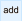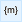﻿ 20-sim webhelp > Language Reference > Keywords > constants

# Constants

Constants are symbolic representations of numerical quantities that do not change during or in between simulation runs. Constants must be defined in 20-sim, using the constants keyword. After the keyword, lines with constant declarations can be entered. Every line must be finished by a semicolon (;). An example is given below:

constants

real V = 1 {volume, m3};                // the volume of barrel 1

real hidden D = 0.5 {length, m};        // the length of part 1

...

## Types

20-sim currently supports four types of constants: boolean, integer, real and string. These types must be specified explicitly. The use of the type real is shown in the example above.

## Hidden

To prevent users from inspecting constants, the keyword hidden can be applied. This keyword should follow the data type.

## Constant names

Constant names can consist of characters and underscores. A constant name must always start with a character. Constant names are case-sensitive. No reserved words may be used for constants. If a reserved word is used, an error message will pop-up while checking the model. In the example above the names V and D are used.

## Constant Values

It is advised to assign every constant a value. Otherwise 20-sim will assign a default value (0, false or an empty string), which can easily lead to misinterpretations. Unlike parameter values, constant values cannot be overridden in the Simulator.

## Quantity and Unit

If a constant is known to represent a physical value, you can define the corresponding quantity and unit. 20-sim will use this information to do a unit check on equations. The use of quantities and units is optional. In the example above the quantities volume and length are used with the units m3 and m.

## Example

constants

real pi = 3.14159265359;

real two pi = 6.28318530718;

integer i = 10 {length,m};                // vehicle length

integer j = 1 {time,s};

constants

string filename = 'data.txt';

integer i = 1;

real state = 0.0;

boolean t = true, f = false;

## Tip

 • You can easily enter constants by using the Add buttonin the Equation Editor Taskbar.
 • You can easily select quantities and units by using the Units buttonof the Equation Editor Taskbar.
 • Use the 20-sim naming convention for contstant names.